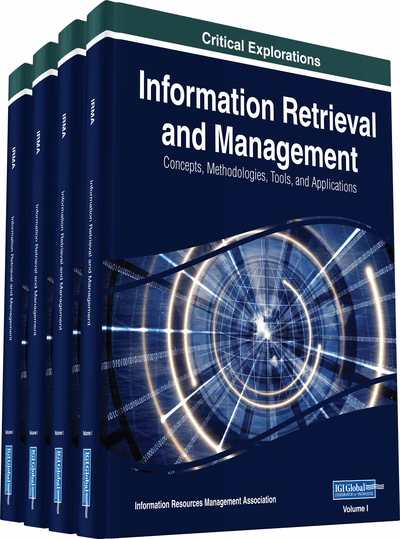# Document Clustering: A Summarized Survey

Harsha Patil (Maulana Azad National Institute of Technology, India) and R. S. Thakur (Maulana Azad National Institute of Technology, India)
DOI: 10.4018/978-1-5225-5191-1.ch003
Available
\$37.50
No Current Special Offers

## Abstract

As we know use of Internet flourishes with its full velocity and in all dimensions. Enormous availability of Text documents in digital form (email, web pages, blog post, news articles, ebooks and other text files) on internet challenges technology to appropriate retrieval of document as a response for any search query. As a result there has been an eruption of interest in people to mine these vast resources and classify them properly. It invigorates researchers and developers to work on numerous approaches of document clustering. Researchers got keen interest in this problem of text mining. The aim of this chapter is to summarised different document clustering algorithms used by researchers.
Chapter Preview
Top

## Introduction

Clustering is the process of subseting data objects. Subsets are based on characteristics of the objects. Objects with similar characteristics are come together and make a set. So, objects from one set have high similarity while objects from other set (C. C. Aggarwalet al, 2012). Example: Suppose we have collection of 10 objects. Here we consider shape characteristic of objects. So objects of same shape are come together in one set. This set is known as cluster and process of dividing similar objects in setwise is known as clustering. After partitioning we get three clusters of objects.

In clustering problem, defining concepts of Optimization criteria is very important. In above example partitioning objects on the basis of their shapes is very easy task, but this is not the always case. In reality, finding the properties of object by which we can make cluster is very difficult, basically it’s very important that objects which belongs to different cluster somehow must be more dissimilar to each other. Suppose, we have collection of n objects lets o1,o2,03..........on. Lets s= {o1,o2,o3......on} and suppose number of clusters are k.so we need to partition n objects into k clusters. That means we need to make k partitions. So suppose those partitions are: P1, P2,P3......Pk. So properties that are shared by these partitions are:

• (i) Pi≠⏀∀i=1,2,3...k

• (ii) Pi∩Pj=⏀∀i≠j

• k

• (iii) ∪Pi=S

• i=1

We need to find some similarity or some disimilarity among objects by which we can partition objects into different sets. Some of widely used similarity and dissimilarity measures are Euclidean distance, Cosine similarity and so on.

### Euclidean Distance

The purpose of a measure of similarity is to compare two lists of numbers (i.e. vectors), and compute a single number which evaluates their similarity. The basis of many measures of similarity and dissimilarity is euclidean distance. The distance between vectors X and Y is defined as follows:

In other words, euclidean distance is the square root of the sum of squared differences between corresponding elements of the two vectors. Note that the formula treats the values of X and Y seriously: no adjustment is made for differences in scale. Euclidean distance is only appropriate for data measured on the same scale. As you will see in the section on correlation, the correlation coefficient is (inversely) related to the euclidean distance between standardized versions of the data.

Euclidean distance is most often used to compare profiles of respondents across variables. For example, suppose our data consist of demographic information on a sample of individuals, arranged as a respondent-by-variable matrix. Each row of the matrix is a vector of m numbers, where m is the number of variables. We can evaluate the similarity (or, in this case, the distance) between any pair of rows. Notice that for this kind of data, the variables are the columns. A variable records the results of a measurement. For our purposes, in fact, it is useful to think of the variable as the measuring device itself. This means that it has its own scale, which determines the size and type of numbers it can have. For instance, the income measurer might yield numbers between 0 and 79 million, while another variable, the education measurer, might yield numbers from 0 to 30. The fact that the income numbers are larger in general than the education numbers is not meaningful because the variables are measured on different scales. In order to compare columns we must adjust for or take account of differences in scale. But the row vectors are different. If one case has larger numbers in general then another case, this is because that case has more income, more education, etc., than the other case; it is not an artifact of differences in scale, because rows do not have scales: they are not even variables. In order to compute similarities or dissimilarities among rows, we do not need to (in fact, must not) try to adjust for differences in scale. Hence, Euclidean distance is usually the right measure for comparing cases.

## Complete Chapter List

Search this Book:
Reset